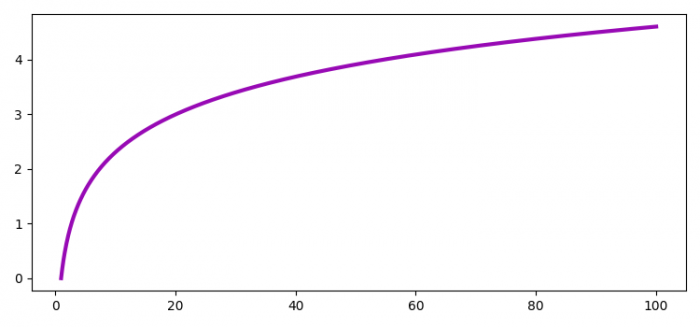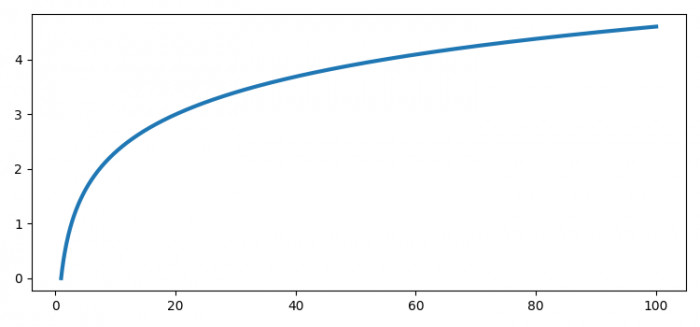# How to avoid line color repetition in matplotlib.pyplot?

To avoid line color repetition in matplotlib.pyplot we can take the following steps −

## Steps

• Set the figure size and adjust the padding between and around the subplots.

• Create x and y data points using numpy.

• Plot the x and y data points using plot() method.

• In the plot() method, use a unique hexadecimal value for the color attribure, for example, color="#980ab5" to set the graph in a unique color. You can also specify a particular color of your choice, for example, color="green".

• To display the figure, use show() method.

## Example

import numpy as np
from matplotlib import pyplot as plt

# Set the figure size
plt.rcParams["figure.figsize"] = [7.50, 3.50]
plt.rcParams["figure.autolayout"] = True

# x and y data points
x = np.linspace(1, 100, 1000)
y = np.log(x)

# Plot the x and y data points with color attribute
plt.plot(x, y, color="#980ab5", lw=3)

# Display the plot
plt.show()

## Output

It will produce the following output −If you run the above code without the color attribute, then the graph will take the default color.